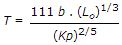# Civil Engineering - Water Resources Engineering

### Exercise :: Water Resources Engineering - Section 2

26.

Time of overland flow, is affected by

 A. slope of the basin B. type of the ground surface C. length of the flow path D. all the above.

Explanation:

No answer description available for this question. Let us discuss.

27.

The rate of rainfall for successive 10 minute periods of a 60 minute duration storm, are shown in the below figure. If the value of φindex is 3 cm/hour, the run off will be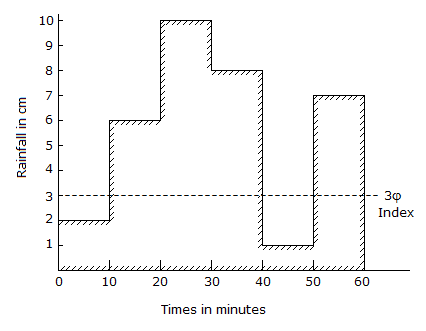A. 2 cm B. 3 cm C. 4 cm D. 5 cm.

Explanation:

No answer description available for this question. Let us discuss.

28.

From the pattern of the rainfall shown in the below figure, the total precipitation isA. 4 cm B. 4.5 cm C. 5 cm D. 5.5 cm E. 6 cm.

Explanation:

No answer description available for this question. Let us discuss.

29.

From the data of the rain storm shown in the below figure, the value of Windex isA. 1.5 cm/hour B. 2 cm/hour C. 2.5 cm/hour D. 2 cm/hour.

Explanation:

No answer description available for this question. Let us discuss.

30.

Izzard formula for the time of concentration in minutes for the plots having no channels, is (where Lo is the length of overland flow in metres and Kp rainfall intensity in cm/hour)

 A.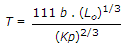B.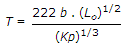C.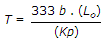D.